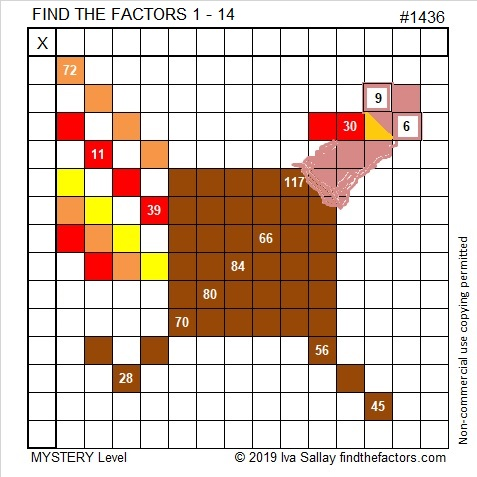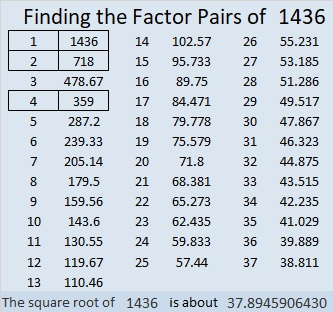# 1436 This Turkey Is Ready

If this year you find yourself needing a bigger turkey, then this one fits that description. This turkey uses math facts from a 14 × 14 multiplication table.

Adding prime number 13 doesn’t cause any problems. You hopefully will recognize that 39 and 119 are both multiples of 13.

However, adding 14 to the puzzle might make you want to call “fowl” because clues 28, 56, 70, and 84 all have two sets of factor pairs where both factors are 14 or less. Can you figure out which clues use 7 and which use 14?

Like always, there is only one solution.Print the puzzles or type the solution in this excel file:  10 Factors 1432-1442

That was puzzle number 1436. Here are a few facts about that number:

• 1436 is a composite number.
• Prime factorization: 1436 = 2 × 2 × 359, which can be written 1436 = 2² × 359
• 1436 has at least one exponent greater than 1 in its prime factorization so √1436 can be simplified. Taking the factor pair from the factor pair table below with the largest square number factor, we get √1436 = (√4)(√359) = 2√359
• The exponents in the prime factorization are 2 and 1. Adding one to each exponent and multiplying we get (2 + 1)(1 + 1) = 3 × 2 = 6. Therefore 1436 has exactly 6 factors.
• The factors of 1436 are outlined with their factor pair partners in the graphic below.1436 is the difference of two squares:
360² – 358² = 1436